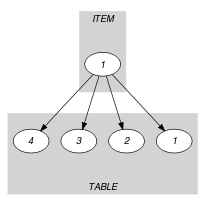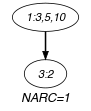## 5.143. element_product

Origin
Constraint

$\mathrm{𝚎𝚕𝚎𝚖𝚎𝚗𝚝}_\mathrm{𝚙𝚛𝚘𝚍𝚞𝚌𝚝}\left(𝚈,\mathrm{𝚃𝙰𝙱𝙻𝙴},𝚇,𝚉\right)$

Synonym

$\mathrm{𝚎𝚕𝚎𝚖𝚎𝚗𝚝}$.

Arguments
 $𝚈$ $\mathrm{𝚍𝚟𝚊𝚛}$ $\mathrm{𝚃𝙰𝙱𝙻𝙴}$ $\mathrm{𝚌𝚘𝚕𝚕𝚎𝚌𝚝𝚒𝚘𝚗}\left(\mathrm{𝚟𝚊𝚕𝚞𝚎}-\mathrm{𝚒𝚗𝚝}\right)$ $𝚇$ $\mathrm{𝚍𝚟𝚊𝚛}$ $𝚉$ $\mathrm{𝚍𝚟𝚊𝚛}$
Restrictions
 $𝚈\ge 1$ $𝚈\le |\mathrm{𝚃𝙰𝙱𝙻𝙴}|$ $𝚇\ge 0$ $𝚉\ge 0$ $\mathrm{𝚛𝚎𝚚𝚞𝚒𝚛𝚎𝚍}$$\left(\mathrm{𝚃𝙰𝙱𝙻𝙴},\mathrm{𝚟𝚊𝚕𝚞𝚎}\right)$ $\mathrm{𝚃𝙰𝙱𝙻𝙴}.\mathrm{𝚟𝚊𝚕𝚞𝚎}\ge 0$
Purpose

$𝚉$ is equal to the ${𝚈}^{th}$ item of $\mathrm{𝚃𝙰𝙱𝙻𝙴}$ multiplied by $𝚇$.

Example
$\left(3,〈6,9,2,9〉,5,10\right)$

The $\mathrm{𝚎𝚕𝚎𝚖𝚎𝚗𝚝}_\mathrm{𝚙𝚛𝚘𝚍𝚞𝚌𝚝}$ constraint holds since its fourth argument $𝚉=10$ is equal to the 3th ($𝚈=3$) item of the collection $〈6,9,2,9〉$ multiplied by $𝚇=5$.

Typical
 $𝚇>0$ $𝚉>0$ $|\mathrm{𝚃𝙰𝙱𝙻𝙴}|>1$ $\mathrm{𝚛𝚊𝚗𝚐𝚎}$$\left(\mathrm{𝚃𝙰𝙱𝙻𝙴}.\mathrm{𝚟𝚊𝚕𝚞𝚎}\right)>1$ $\mathrm{𝚃𝙰𝙱𝙻𝙴}.\mathrm{𝚟𝚊𝚕𝚞𝚎}>0$
Arg. properties
• Functional dependency: $𝚉$ determined by $𝚈$, $\mathrm{𝚃𝙰𝙱𝙻𝙴}$ and $𝚇$.

• Suffix-extensible wrt. $\mathrm{𝚃𝙰𝙱𝙻𝙴}$.

Usage

The $\mathrm{𝚎𝚕𝚎𝚖𝚎𝚗𝚝}_\mathrm{𝚙𝚛𝚘𝚍𝚞𝚌𝚝}$ constraint was originally used in configuration problems [OttossonThorsteinsson00]. In this context, $𝚉$ denotes the cost of buying $𝚇$ units of type $𝚈$ at cost $\mathrm{𝚃𝙰𝙱𝙻𝙴}\left[𝚈\right].\mathrm{𝚟𝚊𝚕𝚞𝚎}$.

Reformulation

By introducing an extra variable $\mathrm{𝚅𝙰𝙻}$, the $\mathrm{𝚎𝚕𝚎𝚖𝚎𝚗𝚝}_\mathrm{𝚙𝚛𝚘𝚍𝚞𝚌𝚝}$$\left(𝚈,\mathrm{𝚃𝙰𝙱𝙻𝙴},𝚇,𝚉\right)$ constraint can be expressed in term of an $\mathrm{𝚎𝚕𝚎𝚖𝚎𝚗𝚝}$$\left(𝚈,\mathrm{𝚃𝙰𝙱𝙻𝙴},\mathrm{𝚅𝙰𝙻}\right)$ constraint and of a product constraint $𝚉=\mathrm{𝚅𝙰𝙻}·𝚇$.

Keywords
Derived Collection
$\mathrm{𝚌𝚘𝚕}\left(\begin{array}{c}\mathrm{𝙸𝚃𝙴𝙼}-\mathrm{𝚌𝚘𝚕𝚕𝚎𝚌𝚝𝚒𝚘𝚗}\left(𝚢-\mathrm{𝚍𝚟𝚊𝚛},𝚡-\mathrm{𝚍𝚟𝚊𝚛},𝚣-\mathrm{𝚍𝚟𝚊𝚛}\right),\hfill \\ \mathrm{𝚒𝚝𝚎𝚖}\left(𝚢-𝚈,𝚡-𝚇,𝚣-𝚉\right)\right]\hfill \end{array}\right)$
Arc input(s)

$\mathrm{𝙸𝚃𝙴𝙼}$ $\mathrm{𝚃𝙰𝙱𝙻𝙴}$

Arc generator
$\mathrm{𝑃𝑅𝑂𝐷𝑈𝐶𝑇}$$↦\mathrm{𝚌𝚘𝚕𝚕𝚎𝚌𝚝𝚒𝚘𝚗}\left(\mathrm{𝚒𝚝𝚎𝚖},\mathrm{𝚝𝚊𝚋𝚕𝚎}\right)$

Arc arity
Arc constraint(s)
 $•\mathrm{𝚒𝚝𝚎𝚖}.𝚢=\mathrm{𝚝𝚊𝚋𝚕𝚎}.\mathrm{𝚔𝚎𝚢}$ $•\mathrm{𝚒𝚝𝚎𝚖}.𝚣=\mathrm{𝚒𝚝𝚎𝚖}.𝚡*\mathrm{𝚝𝚊𝚋𝚕𝚎}.\mathrm{𝚟𝚊𝚕𝚞𝚎}$
Graph property(ies)
$\mathrm{𝐍𝐀𝐑𝐂}$$=1$

Graph model

We use the derived collection $\mathrm{𝙸𝚃𝙴𝙼}$ for putting together the $𝚈$, the $𝚇$ and $𝚉$ parameters of the $\mathrm{𝚎𝚕𝚎𝚖𝚎𝚗𝚝}_\mathrm{𝚙𝚛𝚘𝚍𝚞𝚌𝚝}$ constraint. Within the arc constraint we use the implicit attribute $\mathrm{𝚔𝚎𝚢}$ that associates to each item of a collection its position within the collection.

Parts (A) and (B) of Figure 5.143.1 respectively show the initial and final graph associated with the Example slot. Since we use the $\mathrm{𝐍𝐀𝐑𝐂}$ graph property, the unique arc of the final graph is stressed in bold.

##### Figure 5.143.1. Initial and final graph of the $\mathrm{𝚎𝚕𝚎𝚖𝚎𝚗𝚝}_\mathrm{𝚙𝚛𝚘𝚍𝚞𝚌𝚝}$ constraint(a) (b)
Signature

Because of the first condition of the arc constraint the final graph cannot have more than one arc. Therefore we can rewrite $\mathrm{𝐍𝐀𝐑𝐂}=1$ to $\mathrm{𝐍𝐀𝐑𝐂}\ge 1$ and simplify $\underline{\overline{\mathrm{𝐍𝐀𝐑𝐂}}}$ to $\overline{\mathrm{𝐍𝐀𝐑𝐂}}$.